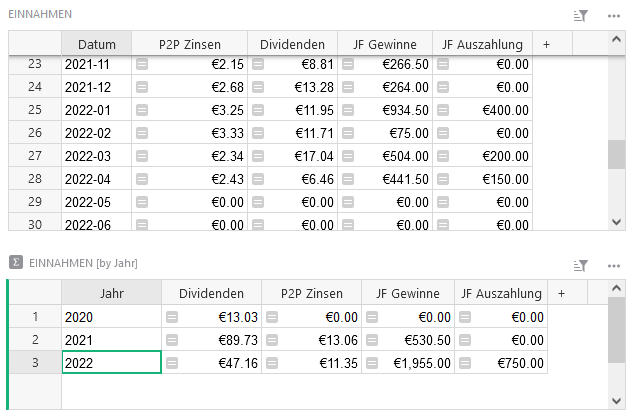# Summary table with content from multiple tables

Hi, I would like to put multiple tables together into an overview summary table.

Details:
Given are 3 tables like

• source_A
• source_B
• source_C

Within each of those tables I have the following columns:
Date (YYYY-MM-DD), Value

How can I create a summary table like:
Date (YYYY-MM) | sum(source_A) | sum(source_B) | sum(source_C)
where the sum only collects the values within the same month of Date(YYYY-MM)?

Based on this summary table, I would then create a bar chart with the month as X value and each source as a series grouped by year.

Thank you!Hi there!

I created an example for you at the link below:

https://public.getgrist.com/eZ8qjxjmCxi7/Community-894/m/fork

First, you’ll need to create helper columns on each of the three tables (Source_A, Source_B and Source_C) that pull the month and year from the Date column. As you see in the three example tables, the Month column has the formula `\$Date.month` and the Year column has the formula `\$Date.year`. This will allow us to pull in values into our Summary table. Once you have these columns set up, feel free to hide them from your table views.

On the summary table, I have a date column using YYYY-MM format then columns for the sum of the orders from the three example tables. The formula used in the Table1 column of the Summary table is:

``````month = \$Date.month
year = \$Date.year
all_orders = Table1.lookupRecords(Month=month, Year=year).Orders
return SUM(all_orders)
``````

First line finds the month from the Date column. Next line finds the year.
The lookupRecords formula finds all records in Table1 where the value in the Month column matches the month value from the first line of this formula and the Year column matches the year value from the second line of this formula.

We use dot notation to pull the values from the Orders column for the records found where month and year match. We assign this list of order values to the variable all_orders.

Last, the formula returns the SUM() of all order values found in the lookupRecords formula.

Change the table name at the beginning of the lookupRecords formula for Table2 and Table3.

Let me know if you have any questions!

1 Like

Thank you @natalie-grist! That was awesome.

My table now displays the expected content.Have a nice weekend!